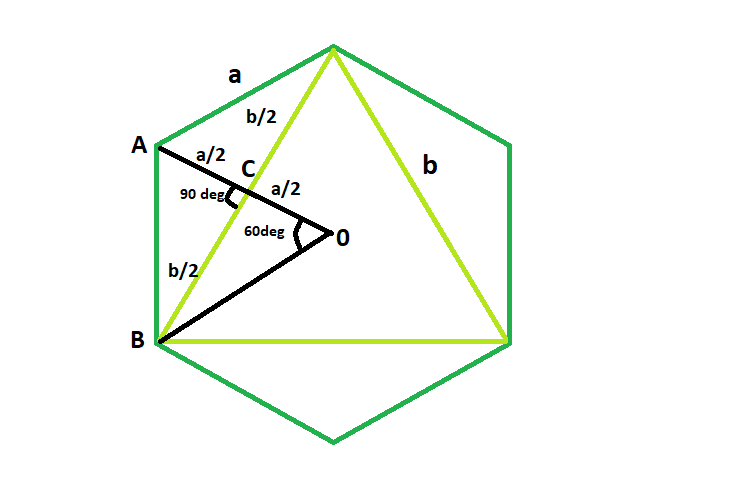GeeksforGeeks App
Open AppBrowser
Continue

# Area of the Largest Triangle inscribed in a Hexagon

Given here is a regular hexagon, of side length a, the task is to find the area of the biggest triangle that can be inscribed within it.
Examples:

```Input:  a = 6
Output: area = 46.7654

Input: a = 8
Output: area = 83.1384```Approach:

It is very clear that the biggest triangle that can be inscribed within the hexagon is an equilateral triangle.
In triangle ACD
following Pythagoras theorem,
(a/2)^2 + (b/2)^2 = a^2
b^2/4 = 3a^2/4
So, b = a√3
Therefore, area of the triangle, A = √3(a√3)^2/4= 3√3a^2/4

Below is the implementation of the above approach:

## C++

 `// C++ Program to find the biggest triangle``// which can be inscribed within the hexagon``#include ``using` `namespace` `std;` `// Function to find the area``// of the triangle``float` `trianglearea(``float` `a)``{` `    ``// side cannot be negative``    ``if` `(a < 0)``        ``return` `-1;` `    ``// area of the triangle``    ``float` `area = (3 * ``sqrt``(3) * ``pow``(a, 2)) / 4;` `    ``return` `area;``}` `// Driver code``int` `main()``{``    ``float` `a = 6;``    ``cout << trianglearea(a) << endl;` `    ``return` `0;``}`

## Java

 `// Java Program to find the biggest triangle``// which can be inscribed within the hexagon` `import` `java.io.*;` `class` `GFG {``    ` `// Function to find the area``// of the triangle``static` `double` `trianglearea(``double` `a)``{` `    ``// side cannot be negative``    ``if` `(a < ``0``)``        ``return` `-``1``;` `    ``// area of the triangle``    ``double` `area = (``3` `* Math.sqrt(``3``) * Math.pow(a, ``2``)) / ``4``;` `    ``return` `area;``}` `    ``public` `static` `void` `main (String[] args) {``        ``double` `a = ``6``;``        ``System.out.println (trianglearea(a));` `    ``}``//This Code is contributed by Sachin..``    ` `}`

## Python3

 `# Python3 Program to find the biggest triangle``# which can be inscribed within the hexagon``import` `math` `# Function to find the area``# of the triangle``def` `trianglearea(a):` `    ``# side cannot be negative``    ``if` `(a < ``0``):``        ``return` `-``1``;` `    ``# area of the triangle``    ``area ``=` `(``3` `*` `math.sqrt(``3``) ``*` `math.``pow``(a, ``2``)) ``/` `4``;` `    ``return` `area;` `# Driver code``a ``=` `6``;``print``(trianglearea(a))` `# This code is contributed``# by Akanksha Rai`

## C#

 `// C# Program to find the biggest triangle``// which can be inscribed within the hexagon` `using` `System;` `class` `GFG {``    ` `// Function to find the area``// of the triangle``static` `double` `trianglearea(``double` `a)``{` `    ``// side cannot be negative``    ``if` `(a < 0)``        ``return` `-1;` `    ``// area of the triangle``    ``double` `area = (3 * Math.Sqrt(3) * Math.Pow(a, 2)) / 4;` `    ``return` `Math.Round(area,4);``}` `    ``public` `static` `void` `Main () {``        ``double` `a = 6;``        ``Console.WriteLine(trianglearea(a));` `    ``}``        ``// This code is contributed by Ryuga` `}`

## PHP

 ``

## Javascript

 ``

Output:

`46.7654`

Time complexity: O(1)

Auxiliary Space: O(1)

My Personal Notes arrow_drop_up